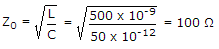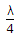# Electronics and Communication Engineering - Microwave Communication

### Exercise :: Microwave Communication - Section 2

46.

A coaxial line has L = 500 nH/m and C = 50 pF/m. The characteristic impedance is

 A. 500 Ω B. 250 Ω C. 100 Ω D. 50 Ω

Answer: Option C

Explanation:.

47.

The main feature of a parametric amplifier is

 A. low noise B. very high gain C. broad bandwidth D. both (b) and (c)

Answer: Option C

Explanation:

Since reactance does not contribute thermal noise to the circuit, it is a low noise device.

48.

Assertion (A): PIN diode is commonly used for microwave control.

Reason (R): A PIN diode uses heavily doped p and n materials.

 A. Both A and R are correct and R is correct explanation of A B. Both A and R are correct but R is not correct explanation of A C. A is correct but R is wrong D. A is wrong but R is correct

Answer: Option B

Explanation:

A PIN diode has an intrinsic (i) layer between p and n layers. When reverse bias is applied depletion layers are formed at p-i and i-n junctions.

The effective/width of depletion layer increases by the width of i layer. It can be used as a voltage controlled attenuator.

At high frequencies the rectification effect ceases and impedance of diode is effectively that of i layer.

This impedance varies with the applied bias. It is used in high frequency switching circuits, limiters, modulators etc.

49.

In a vacuum tube, the transit time of electron between cathode and anode is important at

 A. low frequencies B. high frequencies C. both (a) and (b) D. frequencies which are neither very low nor very high

Answer: Option B

Explanation:

At high frequencies transit time is large as compared to the period of microwave signal.

50.

Assertion (A): A line of lengthand short circuited at far end has an input impedance of infinity.

Reason (R): A line of lengthand short circuited at far end behaves as a parallel resonant circuit.

 A. Both A and R are correct and R is correct explanation of A B. Both A and R are correct but R is not correct explanation of A C. A is correct but R is wrong D. A is wrong but R is correct

Answer: Option A

Explanation:

A parallel tuned circuit has an infinite impedance if R is zero.

#### Current Affairs 2021

Interview Questions and Answers